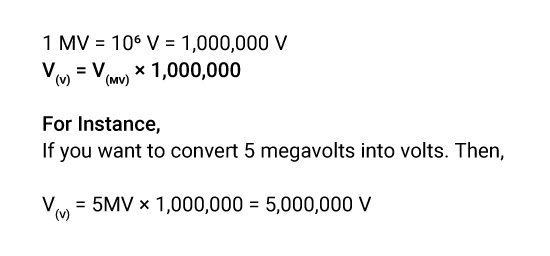## About Our mV to V Converter

“Metric conversion seems fun when you have a handy converter.”

A handy converter can bring you confidence while stuck in a math puzzle. When you use a converter, you don’t need to worry about the units, zeroes and decimals. Whether you want to switch from Millivolts to Volts or looking for another metric conversion, use this tool to save time and get a precise result in a second.

### Definition of Millivolts.

A unit of a potential difference or an electromotive force equal to the one-thousandth of a volt is Millivolt. It is an SI-derived unit of Voltage.

### What is the Symbol of Millivolts?

mV is the symbol of Millivolts.

### How to Convert Millivolts to Volts?

You can convert millivolts to volts by dividing the voltage by the conversion ratio. The voltage in volts is equal to the millivolts divided by one thousand since one volt equals 1000 millivolts.We understand that it is not easy to remember so many formulas. Therefore, we have a converter that remembers every formula and gives you an instant answer. So, millivolts to volts conversion has become way easier.

### How to Use Our Millivolts to Volts Converter?

Convert millivolts to volts in just 3 Simple steps,

• Step2: Put the value of Millivolts that you want to convert.
• Step3: Click “convert” for millivolt to volt conversion.

If you want to amplify millivolts to volts, you are free to use any value you wish using our converter.

### How Many Millivolts Are in a Volt?

1V = 103 mV

1V= 1000 mV

One volt equals one thousand millivolts.

### What is the Difference Between Millivolts to Volts?

Microvolts Volts
A millivolt is a sub-multiple unit of Voltage. Volt is the basic unit of Voltage.
mV represents millivolts. V represents Volts.
One millivolt equals one-thousandth of the volt. One volt equals one thousand millivolts.

### What is the Millivolts Unit of Measure?

Millivolt (mV) measures the electrical potential difference and electromotive force.

### Examples of Converting Millivolts to Volts.

Look at the below examples,

V(V) = 3mV / 1000 = 0.003 V

V(V) = 6mV / 1000 = 0.006 V

V(V) = 7mV / 1000 = 0.007 V

V(V) = 10mV / 1000 = 0.01 V

### Millivolts to Volts Conversion Table.

Here is the standard table for millivolts to volts conversion,

Millivolts Volts
1mV 0.001V
10mV 0.01V
100mV 0.1V
1000mV 1V